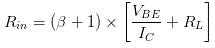# MCQs on Analog Electronics

##### Page 27 of 33. Go to page 1 2 3 4 5 6 7 8 9 10 11 12 13 14 15 16 17 18 19 20 21 22 23 24 25 26 27 28 29 30 31 32 33
01․ The voltage gain of a loaded differential amp is
large than the unloaded voltage gain.
equal to RC / re.
smaller than the unloaded voltage gain.
impossible to determine.

Under construction.

02․ At the unity-gain frequency, the open-loop voltage gain is
1.
AV(mid).
zero.
very large

Under construction.

03․ An emitter follower has a voltage gain that is
much less than one.
approximately equal to one.
greater than one.
zero.

The voltage gain is nothing but the ratio of the output voltage of an amplifier to its input voltage in open circuit condition. An emitter follower has the output voltage that is nearly equal to the input voltage. Hence, the voltage is gain is almost exactly unity.

04․ The input impedance of the base of an emitter follower is usually
low.
high.
shorted to ground.
open.

The input impedance of the emitter follower is given as,Since we know that the β is the current gain of the emitter follower and the current gain is very high in the emitter follower circuit. Hence, the current gain and input impedance are directly proportional to each other. So, the input impedance of an emitter follower is very high.

05․ The AC base voltage of an emitter follower is across the
emitter diode.
DC emitter resistor.
emitter diode and external AC emitter resistance.

The AC base voltage of an emitter follower is approximately equal to the input voltage in between the base and emitter. But in an emitter follower the input voltage is connected to the series combination of base-emitter terminal and the external emitter resistance. The base-emitter junction acts as a diode and this is called as emitter diode. Hence, we know that the total AC base voltage is the summation of the voltage drop across the emitter diode and the external AC emitter resistance.

06․ The output voltage of an emitter follower is across the
emitter diode.
DC collector resistor.
emitter diode and external AC emitter resistance.

In an emitter follower, the load resistance is connected in the output side. So, there is a voltage drop across the load resistance. This voltage drop is nothing but the output voltage of an emitter follower. Hence, it is measured across the load resistance.

07․ The input voltage to an emitter follower is usually
less than the generator voltage.
equal to the generator voltage.
greater than the generator voltage.
equal to the supply voltage.

Due to the source resistance of the emitter follower, there is a small voltage drop across the source resistance. So, rest of the remaining voltage only goes to the input side of emitter follower and hence the input voltage is always less than the generator voltage.

08․ Piezoelectric effect is utilized in which of the following filter?
Pass band.
Band stop.
Ideal filter.
Crystal filter.

A crystal filter refers to a filter produced by using a quartz crystal unit having a stable frequency and steep resonance characteristics relative to the temperature change. Here, the quartz crystal oscillator is worked on the principle of piezoelectric effect. Hence, this piezoelectric effect is utilized in the crystal oscillator.

09․ A transistor has how many PN junctions?
1.
2.
3.
4.

We know that the transistor has three layers. So, in between the two layers, there must be a junction. Hence, the total number of junctions = number of layers ⇒ 1 = 3 - 1 = 2.

10․ What is one important thing transistors do?
Amplify weak signals.
Rectify line voltage.
Step - down voltage.
Emit light.

We know that the transistor can act as an amplifier. The amplifier amplifies the weak signals.

<<<2526272829>>>